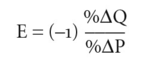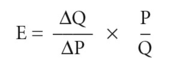# point elasticity

## point elasticity

a precise measure of the responsiveness of DEMAND or SUPPLY to changes in PRICE, INCOME, etc. Taking PRICE-ELASTICITY OF DEMAND, point elasticity may be defined as:where E = price-elasticity of demand, %δQ = percentage change in quantity demanded, P = percentage change in price.

Since the DEMAND CURVE slopes downwards from left to right, the δ Q has the opposite sign to ZiP, so (-1) is added to the equation to generate a positive value for the elasticity figure. The numerical value of E signifies the degree of elasticity, that is, it lies between zero and infinity. An example of the measurement of point elasticity is given in Fig. 143.

In order to measure elasticity at a particular price, P, we need to measure the slope of the demand curve at point T where the demand curve is tangential to the straight line LL. The slope of the tangent LL is equal to δQ/A P where the increment in output (^ Q) is very small. This gives the formula for point elasticity:Where the calculated value of E is greater than 1, demand is described as elastic; where E is less than 1, but greater than zero, demand is inelastic. See also ARC ELASTICITY.

Collins Dictionary of Economics, 4th ed. © C. Pass, B. Lowes, L. Davies 2005
Mentioned in ?
References in periodicals archive ?
If the derivative is presented by first discussing the intuitive motivation behind it, and then the instructor goes through an example where the point elasticity is substantially different from the (easier to calculate) "change" elasticity, students may be encouraged to actually pursue an understanding of the derivative, so that they can have more precise and correct measures in establishing prices for their business.
(1) Phillips (1994, Daellenbach 319) notes the similarity in the results between the two approaches for small changes in price, but asserts that an argument for isoelastic demand curves "is referenced here only for comparison purposes." Apart from the debate, Vazquez (1995) formulates a measure of arc elasticity that is in line with the characteristics of point elasticity and Haque (2005) shows the potential of using arithmetic means to approximate harmonic means in the computation of elasticity.
"We are working on the additional technology that tests point elasticity versus area elasticity," says Chuck Fleishman, chief marketing officer for Southwest Recreational Industries (SRI).
For the point elasticity estimates, data on passenger vehicle ownership in Japan, Republic of Korea, Taiwan, and China were regressed against international dollars per capita GDP levels.
[FIGURE 8 OMITTED] TABLE 1 Regression Results for Point Elasticity Estimates Per Capita Income Range (1990 International Income Adjusted Dollars) Constant Coefficient [R.sup.2] Two-income range model -18.25 2.46 0.96 I < 10,000 (-48.68) (53.96) I [greater than or equal to] 10,000 -10.11 1.61 0.83 (-7.25) (10.99) Four-income range model -15.94 2.15 0.96 I < 5,000 (-30.93) (32.77) 5,000 [less than or equal to] I -18.88 2.53 0.86 < 10,000 (-10.53) (12.58) 10,000 [less than or equal to] I -17.44 2.40 0.78 < 15,000 (-5.82) (7.51) 15,000 [less than or equal to] I -5.84 1.17 0.95 < 20,000 (-6.23) (12.20) Note: t-statistics shown in parentheses.
The above elasticity ranking is based on point elasticity, so the question arises whether other notions of elasticity--in particular, arc elasticity--yield a different interpretation of the price sensitivity of demand in the two markets.
The point elasticity of S with respect to P is given in Equation 9:
The overall elasticity index and the point elasticity can be approximated from unit record household budget data without specifying the form of the concentration curves for budget shares of the commodities under consideration.
[MATHEMATICAL EXPRESSION OMITTED] The point elasticity of the jth commodity evaluated at x can then be expressed in terms of p, [alpha], [beta], [[alpha].sub.j], and [[beta].sub.j] as follows
Gallego-Diaz showed there exists a point on the arc that has a point elasticity equal to that of the isoelastic curve that passes through both a and b.
Whenever two price-quantity situations lie along a curve of constant point elasticity, the arc elasticity of demand shall be equal to that constant value, regardless of the size of the step.
The scale elasticity measure, [Epsilon] = [Delta] ln C/[Delta] ln Q, where C is cost and Q output, is a point elasticity associated with a particular output level and indicates the relative change in cost associated with an incremental change from this output level.

Site: Follow: Share:
Open / Close